kmeans

# Writing A Scikit-Learn Compatible Clustering Algorithm¶

1. Introduction

2. The k-means clustering alogorithm

3. Writing the k-means algorithm with NumPy

4. Writing a Scikit-Learn compatible estimator

5. Using the elbow method and Pipelines

6. Summary & References

## Introduction ¶

Clustering algorithms and unsupervised learning methods have been gaining popularity recently. This is partly because the amount of data being generated has increased exponentially, but also because labels for this data are often still hard to come by. Labeling data can be time consuming and requires human effort which can be expensive. Unsupervised learning methods are machine learning methods that can be used to gleam information from unlabeled data. Clustering algorithms specifically take unlabeled points within a dataset and try to group them into "clusters". Within clusters datapoints are very "similar" (in some sense that will be discussed later) and datapoints between cluster are very "disimilar".

I have mixed feelings on clustering. It's often hard to quantify how well a model is performing when you dont have a measure to define what is correct or not. On the other hand without labeled data, they are often all we've got! Despite being hard to quantify their performance clustering methods can be helpful for Semi-Supervised Learning where there is a small amount of labeled data and a large amount of unlabeled data.

In this post, I will go over how to write a k-means clustering algorithm from scratch using NumPy. The algorithm will be explained in the next section and while seemingly simple, it can be tricky to implement efficiently! As an added bonus, I will go over how to implement the algorithm in a way that is Scikit-Learn compatible so that we can use Scikit-Learn's framework including Pipelines and GridSearchCV (which admittidely isn't particuarly helpful for this model).

Let's start out by talking about the technical details of the k-means clustering algorithm.

## The k-means clustering alogorithm ¶

The k-means clustering algorithm is a means of partitioning a dataset, $\textbf{X} \in \mathbb{R}^{n \times p}$, of $n$ points and $p$ features into $k$ clusters. It specifically assumes

• explicitly that the number of clusters can be defined a-priori.

• implicitly that all points in the dataset belong to clusters that can be well-separated

The main idea of the algorithm is to find a centroid for each of the $k$ clusters, $\boldsymbol \mu_{k} \in \mathbb{R}^{p}$, that represents the "center" of the cluster. The algorithm then assign points within a dataset to the cluster that it is closest to. This assignement requires us to define a metric $d(x_{1}, x_{2})$ to tell the algorithm how close two points are within our feature space or how "similar" they are. Most often the distance function $d$ is taken to the euclidian distance.

The k-means clustering algorithm tries to form clusters which contain points which are similar with respect to the distance metric $d$. It turns out this is equivalent to minimizing the variance within each cluster. Let the set of all clusters be $\mathbf{S} = \{S_1, \, \ldots, \, S_k\}$, then the cost function is defined as,

\begin{align} \mathcal{J}(k) \; &= \; \sum_{j=1}^k |S_j| \operatorname{Var} S_j \\ &= \; \sum_{i=1}^{n} \sum_{j=1}^{k} r_{i,j} \, \Vert \textbf{x}_i - \boldsymbol \mu_j \Vert^{2} \end{align}

Where for each $\textbf{x}_i \in \mathbb{R}^{p}$ we have the definition of the indicator function,

$$r_{i,k} \; = \; \left\{ \begin{array}{cc} 1, & \text{if} \; k = \underset{j} {\operatorname{arg\,min}} \Vert \textbf{x}_j - \boldsymbol \mu_j \Vert^{2} \\ 0, & \text{otherwise} \end{array} \right.$$

It can be seen that the sum, $|S_k| \; = \; \sum_{i=1}^n r_{i,k}$ is the number of points assigned to each cluster!

Minimizing the cost function $\mathcal{J}(k)$ is an NP-hard problem and a heuristic is usually applied to approximate solutions to the optimal centroids. This heuristic is a greedy iterative method that is called the Lloyd–Forgy algorithm and has the following the steps,

1. Intialize the centroids by randomly assigning $\boldsymbol \mu_k$ to one of the datapoints

Then while not converged,

1. Assign each $x_{i}$ to a cluster, i.e. find $r_{i,k}$
2. Update $\boldsymbol \mu_{k}$ by taking them to be the mean of all datapoints in the cluster, \begin{align} \boldsymbol \mu_k \;& = \; \frac{\sum_{i=1}^n r_{i,k} \textbf{x}_i}{\sum_{i=1}^n r_{i,k}} \\ &= \underset{x \in S_k} {\operatorname{avg}}(x) \end{align}

Convergence is usually taken to be that the distance between each cluster's centroid before and after each iteration is less than some predefined tolerance. Another option is that one can also set a maximum number iterations that algorithm can take.

This heuristic method is used to train the k-means model and attempts to find groupings of the data that minimizes the cost function which is the weighted sum of the squares errors of the clusters. Given that is the cost function is a sum of square errors, it is sensitive to outliers just like linear regression. In addition the k-means has the following limitations,

• Requires the number of clusters to be known ahead of time.
• It generally prefers clusters that are approximately the same size.
• Can really only find clusters that are spherical.
• The optimization problem is non-convex and iterative solutions often converge to local optimums instead of global optimum.

Additionally, the algorithm is sensitive to the initial conditions and a number of different random initial conditions are chosen and the one with the best results is chosen as the final model.

### A side note on k-means++¶

The k-means++ is an algorithm for choosing the initial values (or "seeds") for the heuristic solution by specifying a procedure to initialize the cluster centroids before proceeding with the standard iterative k-means algorithm discussed above. The initialization algorithm has the following steps,

• Choose one center uniformly at random among the data points called $\textbf{x}$.
• For each data point $\textbf{x}_{i}$ not chosen yet, compute $d(\textbf{x}_{i}, \textbf{x})$, the distance between $\textbf{x}$ and the nearest center that has already been chosen.
• Choose one new data point at random as a new center, using a weighted probability distribution where a point $\textbf{x}$ is chosen with probability proportional to $d(\textbf{x}_{i}, \textbf{x})^2$.
• Repeat Steps 2 and 3 until $k$ centroids have been chosen.
• Now that the initial centers have been chosen, proceed using standard iterative heuristic.

With the k-means++ initialization, the algorithm is guaranteed to find a solution that is $\mathcal{O}(\log k)$ and is competitive to the optimal k-means solution.

## Writing the k-means algorithm with NumPy ¶

First lets start out by creatinga a simple dataset in 2 dimensions. We can use 10,000 points belonging to 3 clusters as shown below,

In :
import numpy as np
N = 10000
k = 3

data1 = np.random.randn(N//3,2) + np.array([5,6])
data2 = np.random.randn(N//3,2) + np.array([-5,-6])
data3 = np.random.randn(N//3,2) + np.array([-10,3])
data = np.concatenate((data1, data2, data3))


Now we lets plot the points,

In :
import matplotlib.pyplot as plt
%matplotlib inline

plt.scatter(data[:,0], data[:,1])

Out:
<matplotlib.collections.PathCollection at 0x107c81190>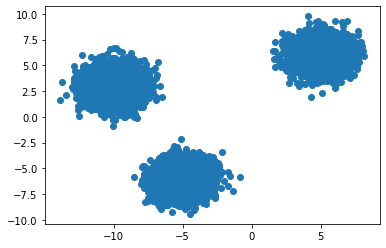### Initializing The Centroids¶

To initialize the centroids we need to come up with a function that takes a dataset of N points and chooses k of them to be the centroids. This is a naive initialization process compared to k-means++, but for our purposes it will do. We define this function using the np.random.choice function to get the entries of the k different points as shown below,

In :
np.random.choice(N, 3,replace=False)

Out:
array([1749, 1121, 1310])

We can then write a function to initialize these centroids,

In :
from typing import List

def init_centroids(X: np.array, K: int) -> List[float]:
N = X.shape
return X[np.random.choice(N, K,replace=False)]

In :
centroids = init_centroids(data, 3)


The three initial points for the centroids are,

In :
centroids

Out:
array([[-11.23745643,   3.46931906],
[ -4.91882472,  -4.87376259],
[  4.78626865,   6.48088   ]])

We can plot these to see where they are with respsect to the rest of the points,

In :
plt.figure()
plt.scatter(data[:,0], data[:,1])
for c in centroids:
plt.scatter(*c, color="red")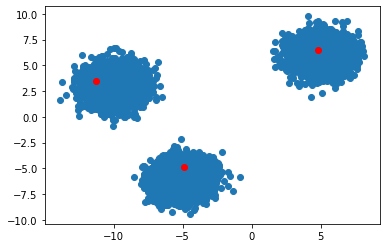Two of the centroids look like they are in the same cluster (note due to random seeding you may not get the same results). Now let's write a function that assigns each of the points in the dataset to a cluster by finding cluster that each point is closest to.

### Assign Each Point To A Cluster¶

Let's take an example with the first data point,

In :
point = data
point

Out:
array([5.24048539, 6.26693666])

We can remind ourselves what the centroids look like,

In :
centroids

Out:
array([[-11.23745643,   3.46931906],
[ -4.91882472,  -4.87376259],
[  4.78626865,   6.48088   ]])

Now we want to find the distance from the point to each of the centroids and will use the concept of broadcasting in NumPy. We can see the shape point and centroids,

In :
print(f"point.shape = {point.shape}")
print(f"centroids.shape = {centroids.shape}")

point.shape = (2,)
centroids.shape = (3, 2)


Now subtracting the two using broadcasting results in

In :
point - centroids

Out:
array([[16.47794182,  2.79761759],
[10.15931011, 11.14069925],
[ 0.45421674, -0.21394334]])

We broadcasted the point from a (2,) shape to a (3,2) to match the shape of the centroids array and then performed elementwise subtraction.

Now we can calculate the distance by using the norm from NumPy's numerical linear alebgra module. The use for the norm allows us to use data from arbitrary dimensions!

In :
dists = np.linalg.norm(point - centroids, axis=1)
dists

Out:
array([16.71374377, 15.07735924,  0.50208027])

Now we assign the point to the cluster which is closes usign the argmin function from NumPy.

In :
np.argmin(dists)

Out:
2

This means point is closest to cluster 3 (size Python is 0 indexed)! We can see this below,

In :
plt.figure()
plt.scatter(data[:,0], data[:,1])
for c in centroids:
plt.scatter(*c, color="red")

plt.scatter(*point, color='purple')

Out:
<matplotlib.collections.PathCollection at 0x107e1f5e0>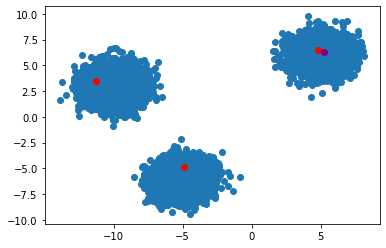Now we want to do this for every point in the dataset, we create a new vector called labels that is the cluster each point belows to. We use the NumPy empty function to assign an empty array of size N (so that the memory is allocated for up front).

In :
labels = np.empty(data.shape)

In :
labels

Out:
array([0., 0., 0., ..., 0., 0., 0.])

Now we can write function to assign all the points in the dataset to the cluster it is closest to. We do this for entire dataset using the enumerate function to keep track of the index in the label array while looping over each point in the dataset,

In :
def update_labels(X: np.array, labels: np.array) -> None:
for i, point in enumerate(X):
dists = np.linalg.norm(point - centroids, axis=1) # norm along the rows
labels[i] = np.argmin(dists)


Note this is function edits the labels array by reference instead of returning a new array.

In :
update_labels(data, labels)
labels

Out:
array([2., 2., 2., ..., 0., 0., 0.])

### Updating The Centroids¶

The last function we need to write is a function that will update the centroids. The way we update the centroids is by finding the points that belong to a each cluster and the finding the mean (or any other average) of those points features to make the new centroid for that cluster.

We can find all the points that belong to each cluster using the concept of Masking. To see how this works we can find all the labeled points that belong to each cluster. For instance we can find which points belong to cluster 0 with the following.

In :
(labels==0)

Out:
array([False, False, False, ...,  True,  True,  True])

Note that this returns a boolean array that says whether each value in the array is a 0 or not. We can then use masking to get all points that are in cluster 0,

In :
data[labels==0]

Out:
array([[-10.49346302,   3.01319403],
[-10.28456084,   2.8061653 ],
[ -9.34551533,   3.14996967],
...,
[-12.78795698,   2.84267242],
[-11.20825349,   4.3302826 ],
[-10.00988014,   4.17066035]])

We can obtain the centroid values by taking the average along each column; this can be calculated with the mean function from NumPy with axis=0 to signify we are summing all the rows in each column,

In :
data[labels==0].mean(axis=0)

Out:
array([2.72674413, 3.4421267 ])

Writing this as a function that takes in the dataframe and labels we can write a for loop over the clusters and then use the stack function to collect the centroids as an array of of centroids.

In :
def update_centroids(X: np.array, labels: np.array, K: int) -> None:
centroids = np.stack([
X[labels==i].mean(axis=0) for i in range(K)
])

return centroids


And can use this to calculate one iteration of the k means algorithm,

In :
update_centroids(data, labels, 3)

plt.figure()
plt.scatter(data[:,0], data[:,1])
for c in centroids:
plt.scatter(*c, color="red")Now, lets right the fit function which will uses all the functions we wrote prior to iteratively fit a k-means model. The last thing we need to mention is convergence which tells us when our iterative method should terminate. The iterative is terminated when,

• We have reached some predefined maximum number of iterations called max_iter
• The distance between the centroids in before and after an iteration is less than some predefined tolerance tol

Note that the method is assumed to have converged only when the second condition is achieved, the former just terminates the iteration since the method has not converged in a reasonable timeframe. The fit is below and returns the centroids of the clusters,

In :
def fit(X: np.array, K: int, max_iters: int = 100, tol:float = 1e-10) -> List[float]:
centroids = init_centroids(X=X, K=K)
labels = np.empty(X.shape)

for _ in range(max_iters):

# label points belonging to clusters
prev_centroids = centroids

# update labels
update_labels(X=X, labels=labels)

# update centroids
centroids = update_centroids(X=X, labels=labels, K=K)

if np.linalg.norm(prev_centroids - centroids) < tol:
break

return centroids


We can use this to now fit out model and verify the results after they have converged,

In :
centroids = fit(X=data, K=3)

plt.figure()
plt.scatter(data[:,0], data[:,1])
for c in centroids:
plt.scatter(*c, color="red")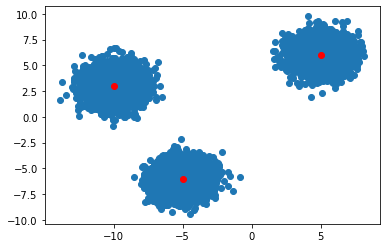Looks pretty good! Now let's talk about how to make this Scikit-Learn compatible.

# Writing a Scikit-Learn compatible estimator ¶

Now we want to go about creating a Scikit-Learn compatible k-means clustering model so that we can use the library's built in Pipeline and GridSearchCV. This realies on our ability to create a custom estimator for Scikit-learn.

In order to accomplish this, we need to create a KMeans clustering class for our model that extends the BaseEstimator class and since this since clustering algorithm we also extend the ClusterMixin class. This method uses the concept of inheritence and abstract base classes or interfaces though not quite so formally.

One thing we change in the implementation is that we allow the number of clusters to be a class member K which is passed to the constructor along with the the maximum number of iteration max_iter, the tolerance tol to determine if the method has converged and a random_state to see the choice of datapoints as initial cluster centroids. The other major changes to the functions written above are mostly either cosmetic or required to make them methods of the class. Specifically we werite the functions to be private methods of the class and therefore require the self parameter and a "_" prefix for all but the fit method which will remain public. Lastly, we change the centroids related methods so that the no longer return the centroids, but rather update the objects centroids member.

The class is written below,

In :
import numpy as np
from sklearn.base import BaseEstimator, ClusterMixin
from __future__ import annotations

class KMeans(BaseEstimator, ClusterMixin):

def __init__(self,
K: int=2,
max_iter: int=10,
random_state: int = 42,
tol: float = 1e-6):

self.K = K
self.max_iter = max_iter
self.centroids = None
self.random_state = random_state
self.tol = tol
np.random.seed(random_state)

def _init_centroids(self, X: np.array) -> None:
N = X.shape
self.centroids = X[np.random.choice(N,self.K,replace=False)]

def _update_labels(self, X: np.array, labels: np.array) -> None:
for i, point in enumerate(X):
dists = np.linalg.norm(point - self.centroids, axis=1) # sum along the rows
labels[i] = np.argmin(dists)

def _update_centroids(self, X: np.array, labels: np.array) -> None:
self.centroids = np.stack([
X[labels==i].mean(axis=0) for i in range(self.K)
])

def fit(self, X: np.array, y: np.array=None) -> KMeans:

self._init_centroids(X)
labels = np.empty(X.shape)

for _ in range(self.max_iter):

# label points belonging to clusters
prev_centroids = self.centroids

# update labels
self._update_labels(X, labels)

# update centroids
self._update_centroids(X, labels)
if np.linalg.norm(prev_centroids - self.centroids) < self.tol:
break

return self

def predict(self, X: np.array) -> np.array:
labels = np.empty(X.shape)
self._update_labels(X, labels)
return labels

def score(self, X: np.array, y: np.array=None) -> np.array:
if y is None:
y = self.predict(X)

variance = np.sum([np.linalg.norm(X[y==i] - self.centroids[i], axis=1).sum()
for i in range(self.K)]) / X.shape
return variance



In addition to the fit function we also have the public method called predict that is required for the BaseEstimator and "Mixin" classes. The predict function predicts which cluster datapoints belong to by calling the _update_labels method under the hood.

In order for our KMeans class to be compatible with the Pipeline and the GridSearchCV we also need to a score method to measure the performance of the object. For our purposes the score function is just the simple sum of square errors.

We can now instantiate a k-means object and fit to the dataset.

In :
kmeans = KMeans(3)

kmeans = kmeans.fit(data)


Note that the fit function returns itself, just as Sciki-learn estimators do!

We can see the centroids,

In :
kmeans.centroids

Out:
array([[-4.99522247, -6.00323426],
[-9.99131782,  3.00841883],
[ 4.98813128,  5.98839757]])
In :
plt.figure()
plt.scatter(data[:,0], data[:,1])
for c in kmeans.centroids:
plt.scatter(*c, color="red")And predict any number of values,

In :
kmeans.predict(np.array([10.3242, 5.321]))

Out:
array([2., 2.])

Lastly we can find the SSE of the model with the score method,

In :
kmeans.score(data)

Out:
1.2630069857409725

# Using the elbow method and Pipelines ¶

We can attempt to compute the appropriate number of clusters a-posterori by using the elbow method over various numbers of $k$.

The idea behind the elbow method is that as the number of clusters increases, the sum of internal variance or SSE decreases rapidly because samples are becoming more and more homogeneous. At a certain point (the elbow point) the clusters contain relatively homogeneous samples and the reduction in SSE is less significant as the number of clusters increases. This inflection point is thought to occur because we are longer seperating legitimate clusters, but rather arbitrarily splitting up clusters and the drop in SSE should be less meaningful.

Let's show how this works on a well-known multi-class dataset where we know the number of cluster beforehand to test to see if the elbow method gives us a reasonable approximation for the number of clusters. We'll use the above dataset which clearly has 3 well-seperated clusters.

Note the clustering is sensitive to scaling and therefore we use the StandardScaler class before applying the clustering model and therefore need to make a pipeline object!

In :
from sklearn.pipeline import make_pipeline
from sklearn.preprocessing import StandardScaler

In :
pipeline = make_pipeline(StandardScaler(), KMeans(K=k))
model = pipeline.fit(data)


We can loop over a number of clusters and get the scores to use the elbow method to determine the number of clusters to use.

In :
K_max = 7
scores = [make_pipeline(StandardScaler(), KMeans(K=k)).fit(data).score(data)
for k in range(1,K_max)]


We can plot the number of clusters vs SSE to find the optimal number of clusters,

In :
plt.plot(range(1,7), scores)
plt.xlabel("# of clustetrs")
plt.ylabel("SSE", rotation=90)
plt.title("Elbo Curve")

Out:
Text(0.5, 1.0, 'Elbo Curve')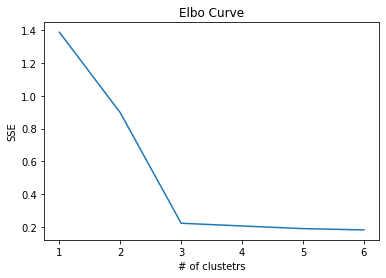Since the 3 clusters are so well separated the elbow is pretty well defined at 3.

Let's take another example where the clusters are not quite so well separated,

In :
new_data = np.concatenate((
np.random.randn(N//3,2) + np.array([3,1]),
np.random.randn(N//3,2) + np.array([-3,0]),
np.random.randn(N//3,2) + np.array([12,5]),
np.random.randn(N//3,2) + np.array([8,8]))
)

plt.scatter(new_data[:,0], new_data[:,1])

Out:
<matplotlib.collections.PathCollection at 0x1262be130>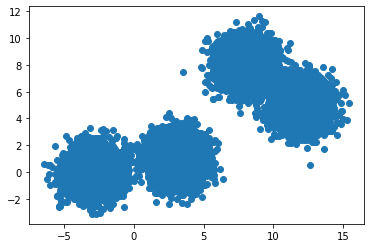This time we have 4 clusters, but two pairs are some what overlapping. We can retrain the k-means model for various values of $K$ and plot the elbow curve again.

In :
K_max = 10
scores = [make_pipeline(StandardScaler(), KMeans(K=k)).fit(new_data).score(new_data)
for k in range(1,K_max)]

plt.plot(range(1,K_max), scores)
plt.xlabel("# of clustetrs")
plt.ylabel("SSE", rotation=90)
plt.title("Elbo Curve")

Out:
Text(0.5, 1.0, 'Elbo Curve')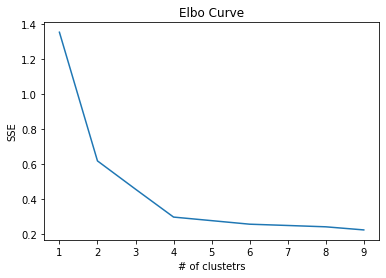The optimal number of clusters could be 2, 3, 4. Obviously the seperating hyperplanes are not as well defined as it was in the prior case!

Lastly let's talk about how to use use the GridSearchCV though again it doesnt make much sense in for unsupersived learning since there is no real metric were trying to optimize. Rather this is just to show the way we created customer estimators and how to use them with the rest of Sciki-learn's tooling.

In :
from sklearn.model_selection import GridSearchCV
from sklearn.pipeline import Pipeline

pipeline = Pipeline([
('scaler',StandardScaler()),
('model', KMeans())
])


Now we can define or grid of cluster sizes and then fit the data,

In :
params = {"model__K": [1,2,3,4,5]}

grid = GridSearchCV(estimator=pipeline,
param_grid=params)

results = grid.fit(data)


And view the best fitting model,

In :
results.best_estimator_

Out:
Pipeline(steps=[('scaler', StandardScaler()), ('model', KMeans(K=1))])

Again the results dont make sense, since grid search is looking for the configuration that maximizes SSE it chooses 1 cluster. However, the main idea is to show how to make a Scikit-Learn compatible estimator!

# Summary & References ¶

In this blogpost we went over how to create a Scikit-learn compatible k-means clustering algorithm using NumPy and the elbow method to go try to determine how many clusters are in our dataset.

I made extensive use of the following references for writing a k-means algorithm from scratch

And Scikit-learn's own documentation to write the Customer Estimator.

I hope you enjoyed this post!